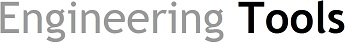Herramientas de Ingeniería

This tool calculates the enthaply of moist air. The enthalpy of moist air is the sum of the enthalpy of the dry air and the enthalpy of the water vapour.

x = Water Weight fraction
(kg/kg)
t = Temperature
( ºC)

The formula used is the following:

h= 1.005 · t + x · (1.88 · t + 2501)

h = Enthapy of the mixture (aire+water vapour) (KJ/Kg)

t = Temperature (ºC)

x = Weight (mass) fraction of water (Kg/Kg) ( 0 < x < 1 )

Enthalpy is the total energy of a thermodynamic system. Enthalpies of ideal gases do not depend on pressure. The unit of measurement in the International System of Units (SI) is the Joule. Specific enthalpy is the enthalpy per kg (kJ/kg) of air.